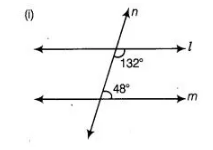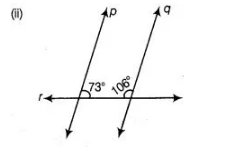# In the figure, which of the two`
Question:

In the figure, which of the two lines are parallel and why?Solution:

In Fig. (i) sum of two interior angles 132° + 48° = 180° [∴ equal to 180°]

Here, we see that the sum of two interior angles on the same side of n is 180°, then they are the parallel lines.

In Fig. (ii), the sum of two interior angles 73° + 106° = 179° ≠ 180°. Here, we see that the sum of two interior angles on same side of r is not equal

to 180°, then they are not the parallel lines.